# Mutual Inductance

Table of Content

## Mutual Inductance

• Consider two coils 1 and 2 placed near each other as shown below in the figure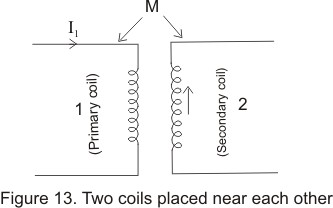• Let coil 1 be the primary coil and coil 2 be secondary coil
• When current is primary coil changes w.r.t time then the magnetic field produced in the coil also changes with time which causes a change in magnetic flux associated with secondary coil
• Due to this change of flux linked with secondary coil an emf is induced in it and this phenomenon is known as mutual induction
• Similarly change in current in secondary coil induces an emf in primary coil.This way as a result of mutual inductance emf is induced in both the coils
• If I1 is the current in primary coil at any instant ,than the emf induced in secondary coil would be proportional to the rate of change of current in primary coil i.e.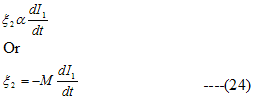Where M is a constant known as coefficient of mutual induction and minus sign indicates that direction of induced emf is such that it opposes the change of current in primary coil
• Unit of mutual inductance is Henry
• We know that a magnetic flux is produced in primary coil due to the flow of current I1.If this is the magnetic flux associated with secondary coil then from faraday's law of EM induction ,emf induced in secondary coil would be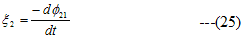• comparing equation (24) and (25) we get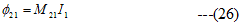Thus coefficient of mutual induction of secondary coil w.r.t primary coil is equal to magnetic flux linked with secondary coil when 1 Ampere of current flows in primary coil and vice-versa
• Similarly ,if I2 is the current in secondary coil at any instant then flux linked with primary coil is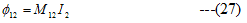where M12 is coefficient of mutual induction of primary coil with respect to secondary coil
• EMF induced in primary coil due to change of this flux is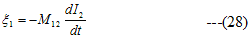• For any two circuits
M12=M21=M
• In general mutual inductance of two coil depends on geometry of the coils (shape ,size, number of turns etc),distance between the coils and nature of material on which the coil is wound

## Mutual Inductance of two co-axial solenoids

• Consider a long solenoid of length l and area of cross-section A containing Np turns in its primary coil
• Let a shorter secondary coil having N2 number of turns be wounded closely over the central portion of primary coil as shown below in the figure.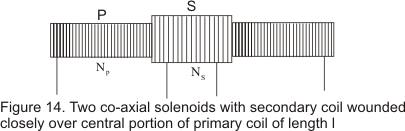• If Ip is the current in the primary coil then magnetic field due to primary coil would be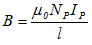• So flux through each turn of secondary coil would be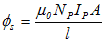where A is the area of cross-section of primary coil
• Total magnetic flux through secondary coil is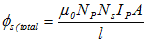• Emf induced in secondary coil is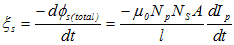Thus from equation 24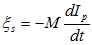So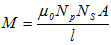## Relation between Mutual inductance and self inductance

• Consider two coils of same length l and same area of cross-section placed near each other as shown below in the figure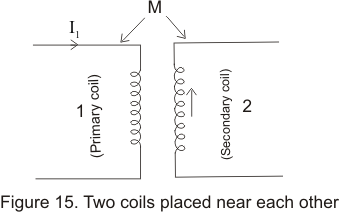• Let there are N1 number of turns in primary coil and N2 number of turns in secondary coil
• A current I1 in the primary coil produces a magnetic field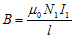which in turns gives rise to flux?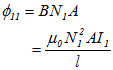in primary coil and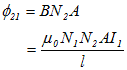in the secondary coil due to current in primary coil.
• By the definition of self induction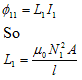and by definition of mutual induction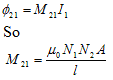• Reversing the procedure if we first introduce the current I2 in secondary coil then we get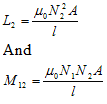• So L1 is the self inductance of primary coil,L2 is the self induction of secondary coil and M21=M12=M is the mutual inductance between two coils

• Product of L1 and L2 is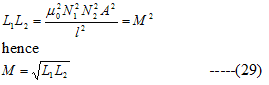• In practice M is always less than eq due to leakage which gives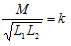Where K is called coefficient of coupling and K is always less then 1.